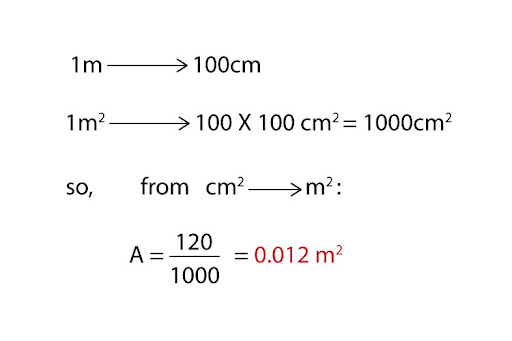# "If a person weighs 400N and the total area of their feet is 120cm^2, what is the pressure they are exerting on the ground?"

A: Pressure is defined as the amount of force exerted on a surface per unit area. Equation-wise, it can be written as follows:Now, we have the person’s weight - i.e. their gravitational force - acting on the ground, through their feet with area 120 cm2. Before we can put in these values in the above equation, we want to first convert the units of area from cm2 to m2:Then, it is just a matter of plugging everything in, and we get our answer!